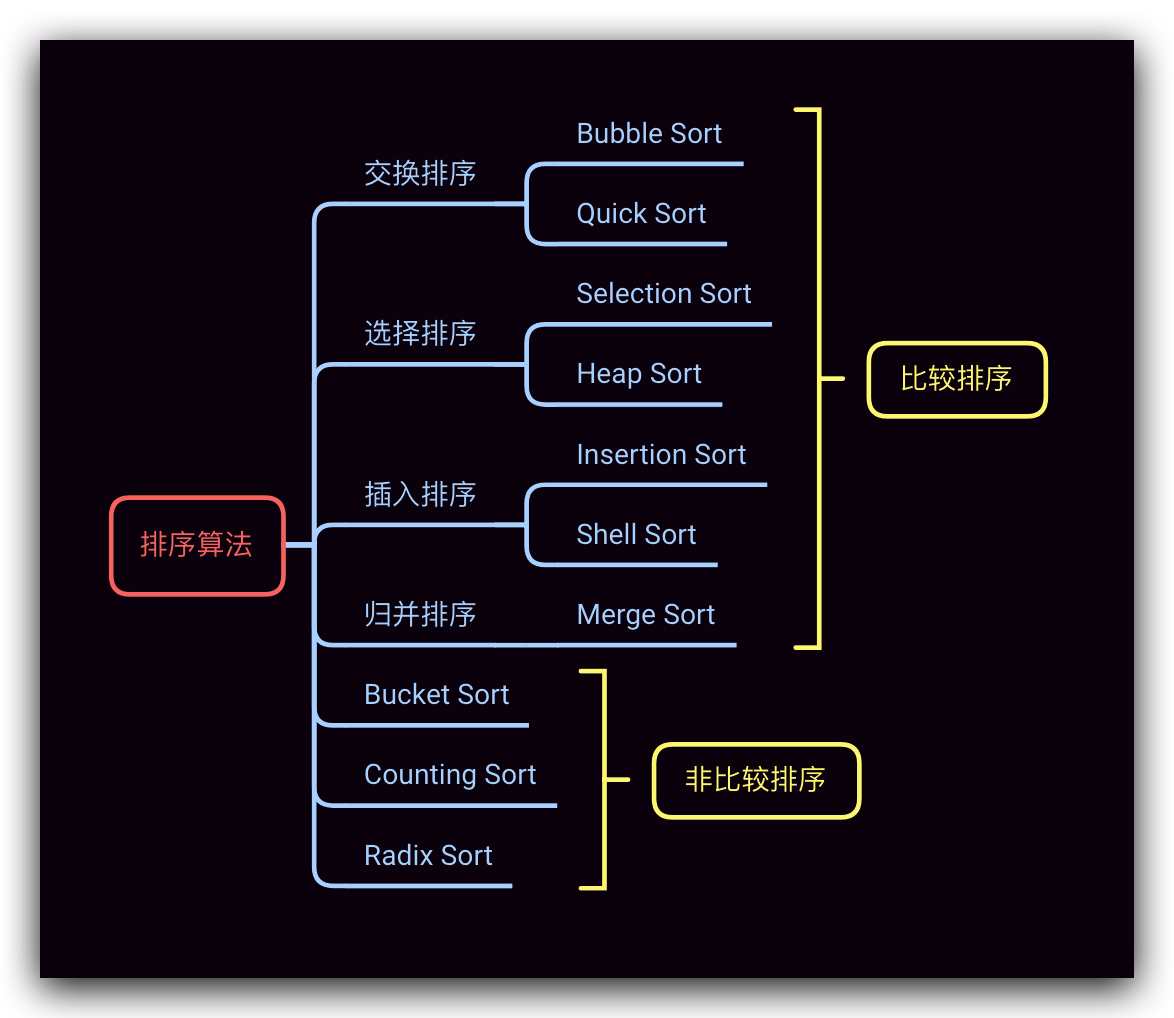# Bubble Sort 冒泡排序

## 算法流程

• 第一趟排序开始时，对所有元素(array～array[N-1])从头到尾依次比较相邻2个元素，如果反序(array[i] > array[i+1])则交换。很显然，第一趟排序结束时，最后一个位置上的元素array[N-1]一定是N个元素中最大的元素，即，array[N-1]排序完成
• 开始下一趟排序，其对剩下尚未排序的元素(array～array[N-2])，从头到尾依次比较相邻2个元素，如果反序则交换
• 冒泡排序中的一趟排序过程可以完成对一个元素的排序，故对于N个元素排序而言，重复上述步骤，当其完成 N-1 趟排序过程后即排序完成

## 算法图解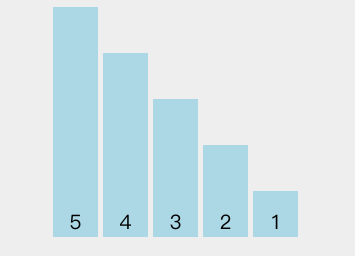## 特点

$O(n^2)$ $O(n^2)$ $O(n)$ $O(1)$ 稳定

## Java 代码示例

Java 代码示例如下：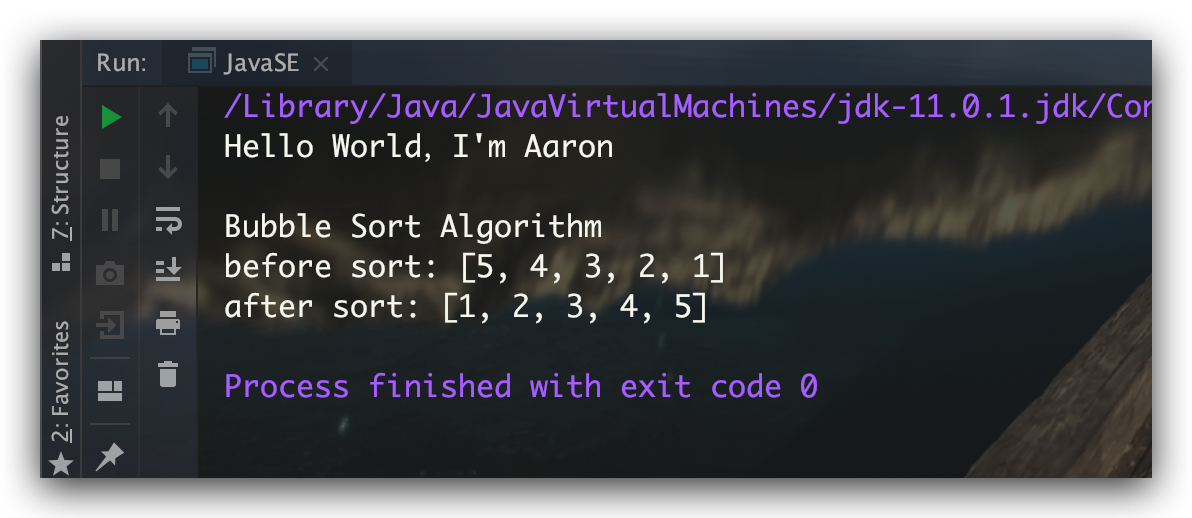# Selection Sort 选择排序

## 算法流程

• 首先，找到数组中最小的元素；然后，将其与数组中的第一个元素交换位置
• 其次，再在剩下待排序的元素中找到最小的元素。然后，将其与数组中的第二个元素交换位置
• 选择排序的一轮”选择-交换”过程，可以完成对一个元素的排序，故对于N个元素排序而言，重复上述步骤，当其完成 N-1 趟排序过程后即排序完成

## 算法图解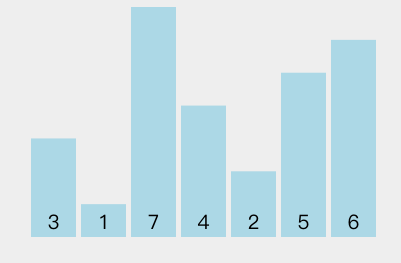## 特点

$O(n^2)$ $O(n^2)$ $O(n^2)$ $O(1)$ 不稳定

## Java 代码示例

Java 代码示例如下：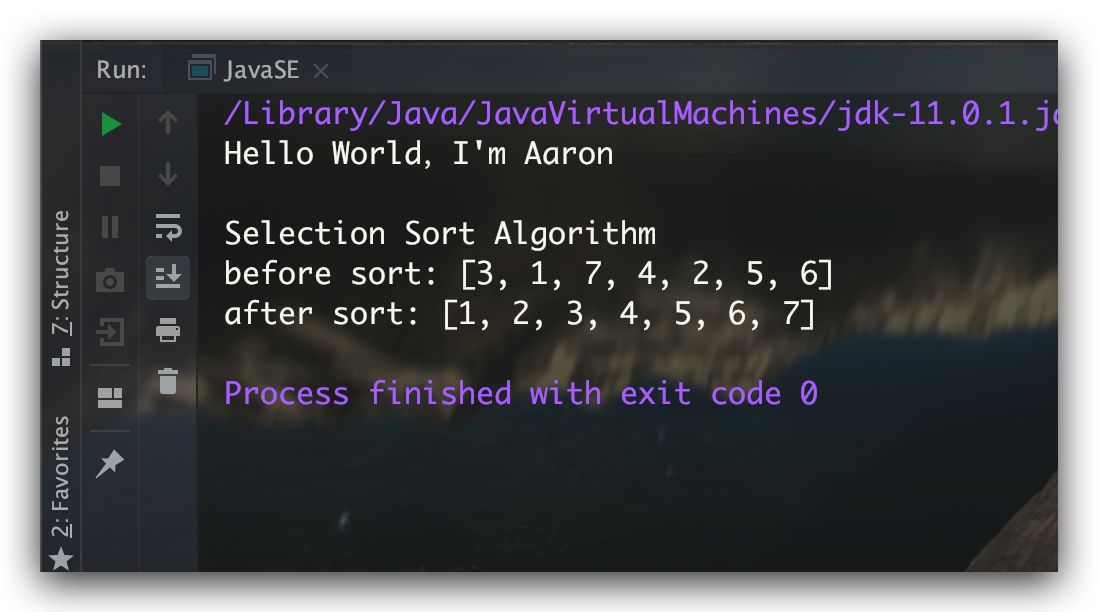# Insertion Sort 插入排序

## 算法流程

• 在插入排序中，索引index左边(=index)的元素则是待插入排序的。故其首先从第二个元素开始插入，即 index=1
• 对于待插入元素array[index]而言，其在左边有序的元素中的位置indexInsert，是最后一个大于array[index]的元素array[indexInsert]
• 在插入元素array[index]时，建议先将 indexInsert及其之后的有序元素 均向后移动一位再插入；而不是两两交换，通过移动的方式将array[index]移动至欲插入位置。此举可以大大提高时间效率
• 重复上述插入过程，直到最后一个元素(index=N-1)插入完毕，即排序完成

## 算法图解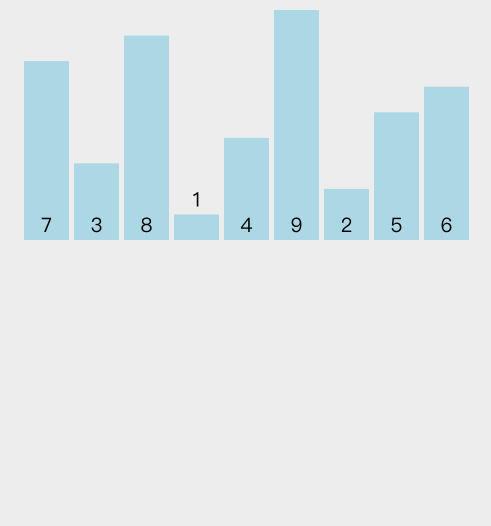## 特点

$O(n^2)$ $O(n^2)$ $O(n)$ $O(1)$ 稳定

## Java 代码示例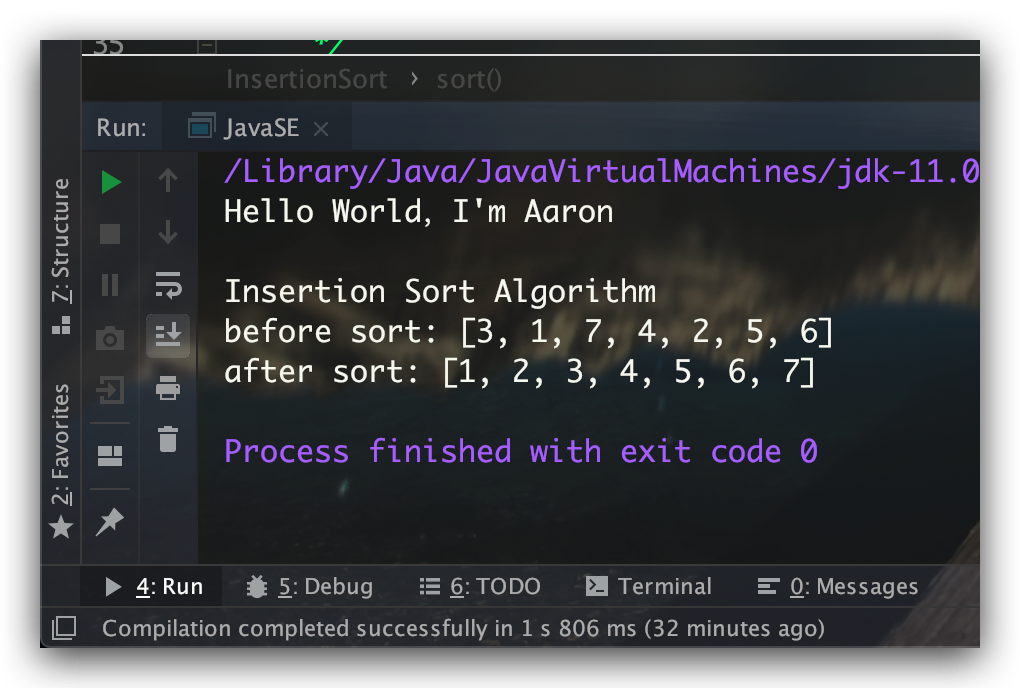# 参考文献

1. 算法(第4版) Robert Sedgewick、Kevin Wayne 著
0%